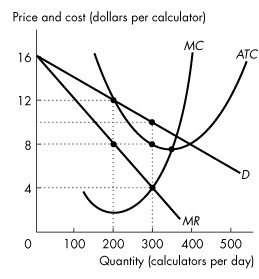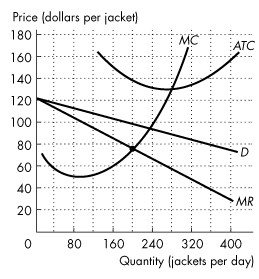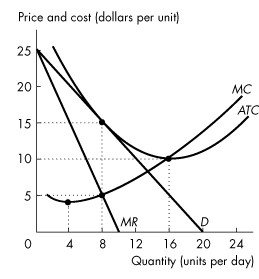/
/
/
51) Which of the following always true regarding a profit-maximizing
Not my Question
Flag Content

# Question : 51) Which of the following always true regarding a profit-maximizing : 1418022

51) Which of the following is always true regarding a profit-maximizing monopolistically competitive firm in short-run equilibrium?

A) P = ATC

B) P = MR

C) MR = MC

D) MC = ATC

E) P = MC

Use the figure below to answer the following questions.Figure 14.2.5

52) Refer to Figure 14.2.5 The figure shows the situation facing Smart Digit, Inc., a firm in monopolistic competition that produces calculators. What is the firm's economic profit per day?

A) zero

B) between \$1 and \$700

C) between \$701 and \$900

D) more than \$901

E) less than zero

53) Refer to Figure 14.2.5. The figure shows the situation facing Smart Digit, Inc., a firm in monopolistic competition that produces calculators. The firm's markup is ________ per calculator.

A) zero

B) \$2

C) \$4

D) \$6

E) \$10

Use the figure below to answer the following questions.Figure 14.2.6

54) Refer to Figure 14.2.6, which shows the demand curve, marginal revenue curve and cost curves faced by Gap. Gap maximizes its profit if it sells ________ jackets per day.

A) 200

B) 240

C) 275

D) 140

E) 280

55) Refer to Figure 14.2.6, which shows the demand curve, marginal revenue curve and cost curves faced by Gap. Gap maximizes its profit if it charges ________ per jacket.

A) \$100

B) \$95

C) \$75

D) \$120

E) \$90

56) Refer to Figure 14.2.6, which shows the demand curve, marginal revenue curve and cost curves faced by Gap. Gap's economic ________ is ________ a day.

A) loss; \$8,000

B) loss; \$13,000

C) profit; \$7,200

D) profit; \$13,000

E) loss; \$8,960

57) Consider a monopolistically competitive industry which is in long-run equilibrium.  Which of the following is true?

A) All firms charge a price equal to average total cost.

B) All firms charge a price equal to marginal cost.

C) All firms make an economic profit.

D) The demand, average total cost, and marginal cost curves all intersect at the same point.

E) Firms have an incentive to enter the industry.

58) In the long-run, a firm in monopolistic competition produces at an output level where

A) P > ATC and MR = MC.

B) P > ATC and MR > MC.

C) P = ATC and MR = MC.

D) P = ATC and MR > MC.

E) P > ATC and MC > ATC.

Use the figure below to answer the following question.Figure 14.2.7

59) Refer to Figure 14.2.7. The figure shows the demand, marginal revenue, and cost curves for a monopolistically competitive firm in the long run. The firm has excess capacity of

A) 4 units.

B) 8 units.

C) 16 units.

D) \$10.

E) \$5.

60) Monopolistic competition might be efficient if

A) firms invested in technology that decreased the marginal cost of production.

B) more firms entered the industry.

C) firms left the industry.

D) the loss that arises because the quantity produced is less than the efficient quantity is offset by the gain that arises from having a greater degree of product variety.

E) firms made more use of brand names.

## Solution 5 (1 Ratings )

Solved
Economics 1 Year Ago 73 Views